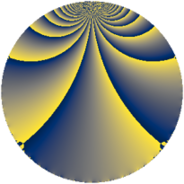# Properties

 Label 9800.2.a.cmLevel $9800$ Weight $2$ Character orbit 9800.a Self dual yes Analytic conductor $78.253$ Analytic rank $1$ Dimension $4$ CM no Inner twists $2$

# Related objects

## Newspace parameters

 Level: $$N$$ $$=$$ $$9800 = 2^{3} \cdot 5^{2} \cdot 7^{2}$$ Weight: $$k$$ $$=$$ $$2$$ Character orbit: $$[\chi]$$ $$=$$ 9800.a (trivial)

## Newform invariants

 Self dual: yes Analytic conductor: $$78.2533939809$$ Analytic rank: $$1$$ Dimension: $$4$$ Coefficient field: $$\Q(\sqrt{2}, \sqrt{5})$$ Defining polynomial: $$x^{4} - 6 x^{2} + 4$$ Coefficient ring: $$\Z[a_1, \ldots, a_{13}]$$ Coefficient ring index: $$2$$ Twist minimal: yes Fricke sign: $$1$$ Sato-Tate group: $\mathrm{SU}(2)$

## $q$-expansion

Coefficients of the $$q$$-expansion are expressed in terms of a basis $$1,\beta_1,\beta_2,\beta_3$$ for the coefficient ring described below. We also show the integral $$q$$-expansion of the trace form.

 $$f(q)$$ $$=$$ $$q + \beta_{1} q^{3} + \beta_{3} q^{9} +O(q^{10})$$ $$q + \beta_{1} q^{3} + \beta_{3} q^{9} - q^{11} + ( \beta_{1} - \beta_{2} ) q^{13} -2 \beta_{1} q^{17} + ( 2 \beta_{1} - \beta_{2} ) q^{19} - q^{23} + ( -2 \beta_{1} + 2 \beta_{2} ) q^{27} + ( -2 - 3 \beta_{3} ) q^{29} + ( -3 \beta_{1} + 3 \beta_{2} ) q^{31} -\beta_{1} q^{33} + ( 2 - \beta_{3} ) q^{37} + 2 q^{39} + ( -3 \beta_{1} - 2 \beta_{2} ) q^{41} + \beta_{3} q^{43} + \beta_{2} q^{47} + ( -6 - 2 \beta_{3} ) q^{51} + ( -1 + \beta_{3} ) q^{53} + ( 5 + \beta_{3} ) q^{57} + ( 3 \beta_{1} + 3 \beta_{2} ) q^{59} + ( -\beta_{1} - 2 \beta_{2} ) q^{61} + ( -3 - 2 \beta_{3} ) q^{67} -\beta_{1} q^{69} + 3 \beta_{3} q^{71} + ( -5 \beta_{1} + 6 \beta_{2} ) q^{73} + ( -3 - 4 \beta_{3} ) q^{79} + ( -4 - 3 \beta_{3} ) q^{81} + ( -3 \beta_{1} - \beta_{2} ) q^{83} + ( -5 \beta_{1} - 6 \beta_{2} ) q^{87} + ( \beta_{1} - 3 \beta_{2} ) q^{89} -6 q^{93} + ( -5 \beta_{1} - \beta_{2} ) q^{97} -\beta_{3} q^{99} +O(q^{100})$$ $$\operatorname{Tr}(f)(q)$$ $$=$$ $$4q + O(q^{10})$$ $$4q - 4q^{11} - 4q^{23} - 8q^{29} + 8q^{37} + 8q^{39} - 24q^{51} - 4q^{53} + 20q^{57} - 12q^{67} - 12q^{79} - 16q^{81} - 24q^{93} + O(q^{100})$$

Basis of coefficient ring in terms of a root $$\nu$$ of $$x^{4} - 6 x^{2} + 4$$:

 $$\beta_{0}$$ $$=$$ $$1$$ $$\beta_{1}$$ $$=$$ $$\nu$$ $$\beta_{2}$$ $$=$$ $$($$$$\nu^{3} - 4 \nu$$$$)/2$$ $$\beta_{3}$$ $$=$$ $$\nu^{2} - 3$$
 $$1$$ $$=$$ $$\beta_0$$ $$\nu$$ $$=$$ $$\beta_{1}$$ $$\nu^{2}$$ $$=$$ $$\beta_{3} + 3$$ $$\nu^{3}$$ $$=$$ $$2 \beta_{2} + 4 \beta_{1}$$

## Embeddings

For each embedding $$\iota_m$$ of the coefficient field, the values $$\iota_m(a_n)$$ are shown below.

For more information on an embedded modular form you can click on its label.

Label $$\iota_m(\nu)$$ $$a_{2}$$ $$a_{3}$$ $$a_{4}$$ $$a_{5}$$ $$a_{6}$$ $$a_{7}$$ $$a_{8}$$ $$a_{9}$$ $$a_{10}$$
1.1
 −2.28825 −0.874032 0.874032 2.28825
0 −2.28825 0 0 0 0 0 2.23607 0
1.2 0 −0.874032 0 0 0 0 0 −2.23607 0
1.3 0 0.874032 0 0 0 0 0 −2.23607 0
1.4 0 2.28825 0 0 0 0 0 2.23607 0
 $$n$$: e.g. 2-40 or 990-1000 Significant digits: Format: Complex embeddings Normalized embeddings Satake parameters Satake angles

## Atkin-Lehner signs

$$p$$ Sign
$$2$$ $$-1$$
$$5$$ $$-1$$
$$7$$ $$1$$

## Inner twists

Char Parity Ord Mult Type
1.a even 1 1 trivial
7.b odd 2 1 inner

## Twists

By twisting character orbit
Char Parity Ord Mult Type Twist Min Dim
1.a even 1 1 trivial 9800.2.a.cm 4
5.b even 2 1 9800.2.a.cn yes 4
7.b odd 2 1 inner 9800.2.a.cm 4
35.c odd 2 1 9800.2.a.cn yes 4

By twisted newform orbit
Twist Min Dim Char Parity Ord Mult Type
9800.2.a.cm 4 1.a even 1 1 trivial
9800.2.a.cm 4 7.b odd 2 1 inner
9800.2.a.cn yes 4 5.b even 2 1
9800.2.a.cn yes 4 35.c odd 2 1

## Hecke kernels

This newform subspace can be constructed as the intersection of the kernels of the following linear operators acting on $$S_{2}^{\mathrm{new}}(\Gamma_0(9800))$$:

 $$T_{3}^{4} - 6 T_{3}^{2} + 4$$ $$T_{11} + 1$$ $$T_{13}^{4} - 6 T_{13}^{2} + 4$$ $$T_{19}^{2} - 10$$ $$T_{23} + 1$$

## Hecke characteristic polynomials

$p$ $F_p(T)$
$2$ $$T^{4}$$
$3$ $$4 - 6 T^{2} + T^{4}$$
$5$ $$T^{4}$$
$7$ $$T^{4}$$
$11$ $$( 1 + T )^{4}$$
$13$ $$4 - 6 T^{2} + T^{4}$$
$17$ $$64 - 24 T^{2} + T^{4}$$
$19$ $$( -10 + T^{2} )^{2}$$
$23$ $$( 1 + T )^{4}$$
$29$ $$( -41 + 4 T + T^{2} )^{2}$$
$31$ $$324 - 54 T^{2} + T^{4}$$
$37$ $$( -1 - 4 T + T^{2} )^{2}$$
$41$ $$4 - 94 T^{2} + T^{4}$$
$43$ $$( -5 + T^{2} )^{2}$$
$47$ $$( -2 + T^{2} )^{2}$$
$53$ $$( -4 + 2 T + T^{2} )^{2}$$
$59$ $$324 - 126 T^{2} + T^{4}$$
$61$ $$100 - 30 T^{2} + T^{4}$$
$67$ $$( -11 + 6 T + T^{2} )^{2}$$
$71$ $$( -45 + T^{2} )^{2}$$
$73$ $$1444 - 174 T^{2} + T^{4}$$
$79$ $$( -71 + 6 T + T^{2} )^{2}$$
$83$ $$100 - 70 T^{2} + T^{4}$$
$89$ $$100 - 30 T^{2} + T^{4}$$
$97$ $$1444 - 174 T^{2} + T^{4}$$